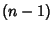## Surface of Section

A surface (or ``space'') of section is a way of presenting a trajectory in-D Phase Space in an-D Space. By picking one phase element constant and plotting the values of the other elements each time the selected element has the desired value, an intersection surface is obtained. If the equations of motion can be formulated as a Map in which an explicit Formula gives the values of the other elements at successive passages through the selected element value, the time required to compute the surface of section is greatly reduced.